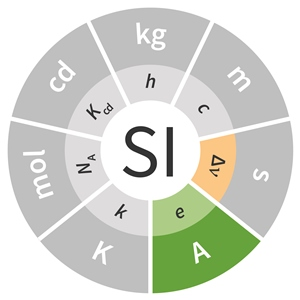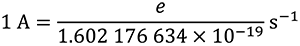# SI base unit: ampere (A)

The ampere, symbol A, is the SI unit of electric current. It is defined by taking the fixed numerical value of the elementary charge e to be 1.602 176 634 x 10–19 when expressed in the unit C, which is equal to A s, where the second is defined in terms of ΔνCs.This definition implies the exact relation e = 1.602 176 634 x 10–19 A s. Inverting this relation gives an exact expression for the unit ampere in terms of the defining constants e and ΔνCs:which is equal toThe effect of this definition is that one ampere is the electric current corresponding to the flow of 1/(1.602 176 634 x 10–19) elementary charges per second.